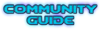## Simple glow shader for HUD elements

### Simple glow shader for HUD elements

This is a shader meant for hud graphics that are supposed to glow. The larger you want your glow to be, the more transparent space you'll need to have around the edge of your graphic.The armor pieces on the little dude at the left edge of the screen shows the effect.

Code: Select all
`//texture glow fragment shader by kodi//use as you wish, no need to ask. Credits appreciated though!//control the shader with these. Both radialSamples and size affect performance. The former should not be a lower value than the latter.const int radialSamples = 16;const int size = 8;const float intensity = 0.005;//full revolution in radiansconst float twopi = 6.2831;vec4 Process(vec4 color) {   vec2 tc = gl_TexCoord.st; //coordinate of the fragment(pixel) in the image   vec4 color1 = getTexel(tc); //texture color of fragment   ivec2 texSize = textureSize(tex, 0); //texture size in pixels   vec2 pixel = 1.0/vec2(texSize); //pixel size in a normalized 0-1.0 range   vec4 newcolor = color1;   if(color1.a <= 0) //if the fragment is transparent we attempt adding glow   {      for(int i=1; i<=radialSamples; i++) //in this loop, we rotate around the fragment to check color of neighbouring ones      {         float angle = twopi*(float(i)/float(radialSamples)); //angle, increases each time "i" does.          vec2 offset = vec2(cos(angle)*pixel.x,sin(angle)*pixel.y); //gets offset to a pixel adjacent to the original         for(int j=1; j<=size; j++) //in this loop, we check the color of (size) amount of pixels in a straight line         {            vec4 glowSample = getTexel(tc+(offset*float(j))); //gets the color of a new sample               newcolor.rgb = newcolor.rgb + (glowSample.rgb*intensity);  //adds to the original            newcolor.a = min(1.0, newcolor.a+(glowSample.a*intensity)); //adjusts and caps alpha         }      }   }   return newcolor;}`kodi
ProscrastinatorJoined: 06 May 2013